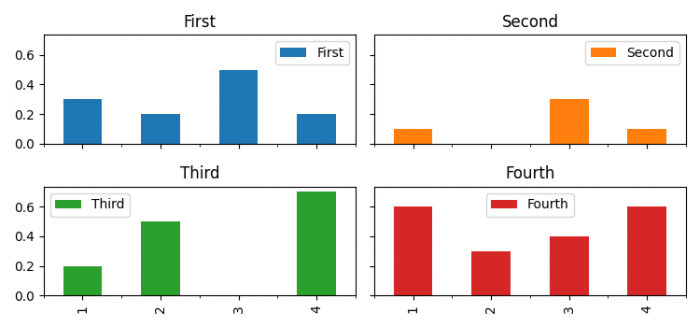# How to add a shared x-label and y-label to a plot created with Pandas' plot? (Matplotlib)

To add a shared x-label and shared y-label, we can use plot() method with kind="bar", sharex=True and sharey=True.

## Steps

• Set the figure size and adjust the padding between and around the subplots.
• Create a two-dimensional, size-mutable, potentially heterogeneous tabular data.
• Plot the dataframe with kind="bar", sharex=True and sharey=True.
• To display the figure, use show() method.

## Example

import pandas as pd
import matplotlib.pyplot as plt

plt.rcParams["figure.figsize"] = [7.50, 3.50]
plt.rcParams["figure.autolayout"] = True

df = pd.DataFrame(
{'First': [0.3, 0.2, 0.5, 0.2], 'Second': [0.1, 0.0, 0.3, 0.1],
'Third': [0.2, 0.5, 0.0, 0.7], 'Fourth': [0.6, 0.3, 0.4, 0.6]},
index=list('1234'))

axes = df.plot(kind="bar", subplots=True, layout=(2, 2),
sharey=True, sharex=True)

plt.show()

## Output